This is a child page. You can use Parent in the quick nav bar at the top or the bottom of the page to navigate directly back to the parent of this page. Some child pages are more than one level deep, and in that case will require more than one Parent click to reach the outermost document level.

### § 16.96.20 - Scripting Examples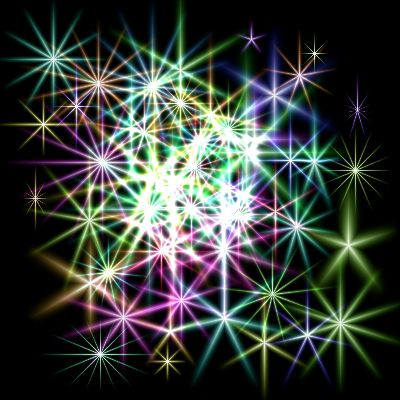Saturated Random Asterizations Script Results

#### § 16.96.20.1 - Simple Random Asterizations

This script allows you to create asterizations in unpredictable colors all over the colorspace.

10 Z=ASTERIZE(rnd(0)*255,rnd(0)*255,rnd(0)*255,0,WID,0,ARMS,0,1,1)

#### § 16.96.20.2 - Saturated Random Asterizations

This script creates only colors that exist around the outer edge of the color wheel; that is, they are always fully saturated colors.

10 REM lines 100-120 assign values for the asterize parameters we'll be changing 20 REM R, G and B are calculated as zero through one (you'll see why in a minute) 30 REM lines 130 through 150 locate the minimum R, G or B value 40 REM lines 160 through 180 move r g and b down so that the minimum value becomes zero 50 REM lines 190 through 210 locate the maximum R, G or B value 60 REM lines 220 through 240 scale R, G and B so that the maximum R, G or B value is 255 70 REM line 250 executes the asterize operation - with fully saturated random colors! 80 REM You can pass S out as the center saturation too, for fun 100 R=RND()*1: G=RND()*1: B=RND()*1: S=RND()*100 110 ARMS=(RND()*13)+4 120 WID = (RND()*20)+10 130 MI = R 140 IF G<MI THEN MI = G 150 IF B<MI THEN MI = B 160 R = R-MI 170 G = G-MI 180 B = B-MI 190 MA = R 200 IF G>MA THEN MA = G 210 IF B>MA THEN MA = B 220 R = (R/MA)*255 230 G = (G/MA)*255 240 B = (B/MA)*255 250 Z=ASTERIZE(R,G,B,0,WID,0,ARMS,0,1,1) 260 REM Z=ASTERIZE(R,G,B,S,WID,0,ARMS,0,1,1)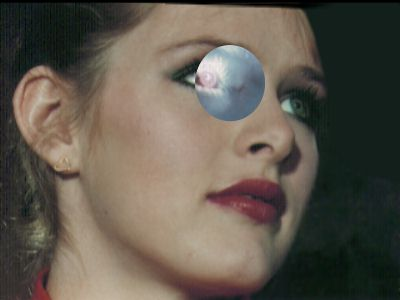Custom Negative Operator Script Results

#### § 16.96.20.3 - Image Negative Operator — Written entirely in the WIS scripting language

10 REM Demonstrates how to process an area selection - color negative function 20 X1=GETX1(0): Y1=GETY1(0): X2=GETX2(0): Y2=GETY2(0) 30 FOR Y=Y1 TO Y2 40 A=PROGRESS(Y-Y1): IF A=1 THEN END 50 FOR X=X1 TO X2 60 R=255-GETRED(X,Y): G=255-GETGREEN(X,Y): B=255-GETBLUE(X,Y) 70 A=PUTRED(X,Y,R): A=PUTGREEN(X,Y,G): A=PUTBLUE(X,Y,B) 80 NEXT X 100 NEXT Y

If you look this script over, you'll see it is processing every pixel in a rectangle described by X1, Y1, X2 and Y2, values it retrieves from WinImages F/x. But in the example image, the effect is round; what's going on?

The answer is easy: When you make your area selection, F/x creates a mask that limits the application of changes to the selected area. Technically speaking, this means that although you process the entire rectangle that contains the area selection, F/x prevents your changes from being applied anywhere outside the actual selection shape, which is often not rectangular at all.

This very simple mechanism allows you to concentrate on figuring out more generally what you want to do to the image pixels, while F/x takes care of almost all the area selection issues for you; all you need to do with regard to area selections is process a rectangle exactly as we show you here.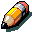Note:

If antialiasing and/or transparency is in use, then portions of the area selection will be "soft" in some places, and the pixel information you send to F/x will be mixed with the original image information according to how soft the area selection mask is.

You can (and should!) use the image negative example here to create other image operations.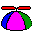Tip:

F/x does not use the mask to limit the pixel information you can read from the image, so you can write image processes that use pixel information outside the masked area to make computations.

Some scripts require access to an unchanged copy of the image in order to read information surrounding the pixel to be processed; if you fetch the surrounding pixels from the image you are changing, early changes affect later computations. Many filters are subject to this processing issue.

This task is easily addressed in the .WIS scripting language. When the user makes an area selection and the Scripting operator is the one in use, F/x will take a complete copy of the image and place it in the undo buffer before it calls your script. You can then use the GETREDU(), GETGREENU(), GETBLUEU() and GETALPHAU() functions to retrieve the original, unchanged image data from the undo buffer all during the time you are changing the actual image. Problem solved!

Here's a second example; this one uses the get and put hue functions to make the image greener. Otherwise, it is essentially the same as the negative example.

10 REM Demonstrates how to process an area selection - greener hue function 20 X1=GETX1(0): Y1=GETY1(0): X2=GETX2(0): Y2=GETY2(0) 30 FOR Y=Y1 TO Y2 40 A=PROGRESS(Y-Y1): IF A=1 THEN END 50 FOR X=X1 TO X2 60 H=GETHUE(X,Y)+20 70 A=PUTHUE(X,Y,H) 80 NEXT X 100 NEXT Y

#### § 16.96.20.4 - How to process a PaletteBang palette, prior to inversion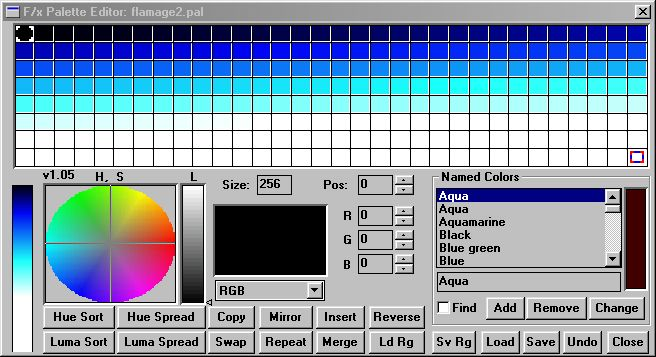Bang palette, inverted
110 REM This Inverts the BANG palette 120 A\$ = "bang" 130 S = GETPALSIZE("BANG")-1 140 IF S > 0 THEN GOTO 170 150 PRINT "WHOOPS! ";A\$;" HAS NO SIZE!" 160 END 170 FOR I=0 TO S 180 R=GETPALETTE(A\$,I,"R") 190 G=GETPALETTE(A\$,I,"G") 200 B=GETPALETTE(A\$,I,"B") 210 R = 255-R 220 G = 255-G 230 B = 255-B 240 E=SETPALETTE(A\$,I,"R",R) 250 E=SETPALETTE(A\$,I,"G",G) 260 E=SETPALETTE(A\$,I,"B",B) 270 NEXT I

or, more compactly...

#### § 16.96.20.5 - How to process a Palette, reprised

110 REM This Inverts the BANG palette 120 A\$ = "bang" 130 S = GETPALSIZE("BANG")-1 140 IF S > 0 THEN GOTO 170 150 PRINT "WHOOPS! ";A\$;" HAS NO SIZE!" 160 END 170 FOR I=0 TO S 180 R=SETPALETTE(A\$,I,"R",255-GETPALETTE(A\$,I,"R")) 190 G=SETPALETTE(A\$,I,"G",255-GETPALETTE(A\$,I,"G")) 200 B=SETPALETTE(A\$,I,"B",255-GETPALETTE(A\$,I,"B")) 210 NEXT I

#### § 16.96.20.6 - How to process a Profile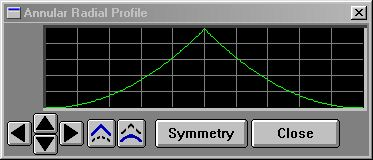Annular Profile, prior to adding ten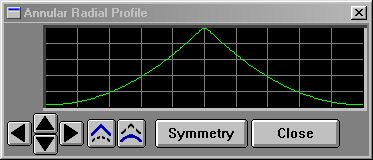Annular profile, with 10 added to every position
10 REM Adds 10 to the named profile curve 20 A\$="annular" 30 for i=0 to 200 40 d=getprof(A\$,i) 50 d = d + 10 60 if d>255 then d=255 70 e=setprof(A\$,i,d) 80 next i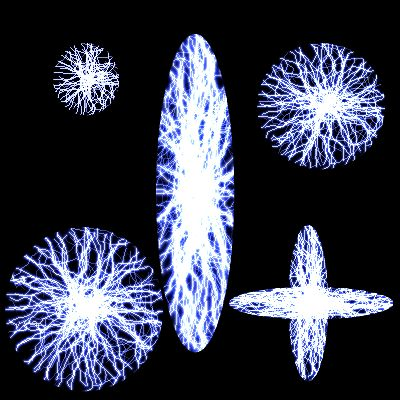Multi-bolt Script Results

#### § 16.96.20.7 - Multi-bolt Lightning Effect

This one is simple, but uses a little trick. You'll see that we're creating an angle that steps from 0 to 350; that accounts for the lightning bolt going across the selection at all angles. But we're passing in the angle (A) as the random number seed too — what that does is ensures that each bolt is different from the others. We don't go to 360, because rotationally speaking, 360 is the same as 0 and that means we'd shoot a bolt twice at the same place.

10 REM multi-bolt lightning effect 20 FOR A=0 TO 350 STEP 10 30 B=LIGHTNING(5,A,10,A,50,5,30,255,20,25,0,1) 100 NEXT A

#### § 16.96.20.8 - Gold Foil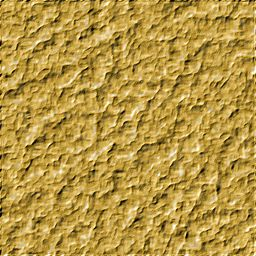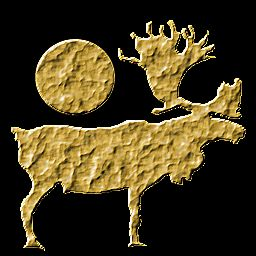This script will create a gold foil texture.

10 r=128:g=128:b=128 20 a=colorfill(r,g,b): REM basic middle grey to texturize 30 x=1:y=1:amt=100:luma=1 40 a=dither(x,y,amt,RND()*100,luma): REM make fine grain texture 50 x=8:y=8:amt=100 60 a=dither(x,y,amt,RND()*100,luma): REM add large grain to texture 70 radi=33 80 a=blur(radi): REM wear the edges off the texture 90 channel=5 100 a=minimum(channel,0): REM expand the holes in the texture 110 amt=4 120 a=relief(amt): REM give it highlights and shadows 130 h=43:s=54:l=0:appsat=1 140 a=colorize(h,s,l,appsat): REM turn it an aged gold color 150 amt=100:radi=20 160 a=sharpen(amt,radi) 170 x=3:y=3:amt=22 180 a=dither(x,y,amt,RND()*100,luma): REM give it some reflectivity 190 end

#### § 16.96.20.9 - Camera Flare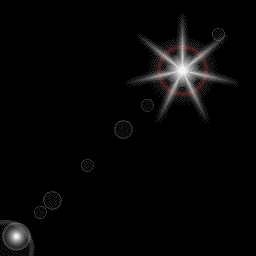Camera Flare Script Results

This is a great example of a more advanced script. This uses annular rings and an asterism to produce a very convincing "camera flare" effect. The effect follows a freehand area selection from the start point to the end point, and so can be made to track with bright spots and camera angles just as a real flare does - as shown in the example animation above.

5 REM this script creates a camera flare using annular ring and asterize 6 REM along with the freehand area selection tool (input from the user) and 7 REM a special elliptical area selection function. This doesn't set the 8 REM asterize profile because the default works well. For WinImages F/x R7. 10 REM general setup 20 a\$="annular": REM this is the profile we'll need to change 30 pi = 3.141592653 40 xw = 30: REM xw and yw define the general size of the effects used 50 yw = 30 60 am = 1: REM am=1 means effects will be additive 70 REM get the endpoints of the line the user has drawn with the freehand tool 80 x1 = GETLI(0): y1 = GETLI(1): x2 = GETLI(2): y2 = GETLI(3) 90 REM p is the position along the line; siz is the size of the effect; peak is intensity 100 REM here are the mild rings 110 peak=50: gosub 6000: r = 255: g = 255: b = 255 111 p = 0.0: siz = 1.0: gosub 5000 120 p = .05: siz = .50: gosub 5000 130 p = .15: siz = .25: gosub 5000 140 p = .20: siz = .35: gosub 5000 150 p = .35: siz = .25: gosub 5000 160 p = .50: siz = .35: gosub 5000 170 p = .60: siz = .25: gosub 5000 180 p = .90: siz = .25: gosub 5000 190 REM here is the big flat glare 200 peak=25: gosub 7000: p=.75: siz=1.25: gosub 5000 210 REM here is the red ring 220 peak=50: gosub 8000: p=.75: g=0: b=0: siz=.90: gosub 5000 230 REM here is the little hotspot 240 peak=255: gosub 9000 250 p=.05: g=255: b=255: siz=.5: gosub 5000 260 REM asterize hot spot effect 270 cs=0: wid=20: rot=TLPOS(360): arms=7: p=.75: siz=2 280 x = x1 + ((x2-x1) * p): y = y1 + ((y2-y1) * p) 290 a=SELOVAL(x,y,xw*siz,yw*siz) 300 a=ASTERIZE(r,g,b,ccs,wid,rot,arms,1,0,am) 310 end 5000 REM draws an annular ring at position p along the line in rgb and profile 5010 x = x1 + ((x2-x1) * p): y = y1 + ((y2-y1) * p) 5020 a=SELOVAL(x,y,xw*siz,yw*siz) 5030 a=ANNULAR(r,g,b,0,am) 5040 return 6000 REM flat 75%, then hump to peak and back to zero profile subroutine 6010 l=peak * .25 6020 REM peak=200 6030 for ii=0 to 150 6040 a=SETPROF(a\$,ii,l) 6050 next ii 6060 s=151: e=200 6070 for ii=s to e 6080 j=(ii-s)/(e-s) 6090 k=abs(sin(j*pi))*peak 6100 if j > .5 then goto 6120 6110 if k < l then k=l 6120 a=SETPROF(a\$,ii,k) 6130 next ii 6140 return 7000 REM flat,then fall-off profile subroutine 7010 REM peak=64 7020 s=150: e=200 7030 for ii=0 to s-1 7040 a=SETPROF(a\$,ii,peak) 7050 next ii 7060 for ii=s to e 7070 j = 1-((ii-s)/(e-s)) 7080 a=SETPROF(a\$,ii,peak*j) 7090 next ii 7100 return 8000 REM bright ring profile subroutine 8010 s=150: e=200 8020 REM peak=200 8030 for ii=0 to s 8040 a=SETPROF(a\$,ii,0) 8050 next ii 8060 for ii=s to e 8070 j=(ii-s)/(e-s) 8080 k=abs(sin(pi*j)) 8090 a=SETPROF(a\$,ii,k*peak) 8100 next ii 8110 return 9000 REM burst profile subroutine 9010 s=0: e=200 9020 for ii=s to e 9030 j=1-abs(sin(((ii/200)/2)*pi)) 9040 a=SETPROF(a\$,ii,j*peak) 9050 next ii 9060 return

#### § 16.96.20.10 - Setting Profiles via Scripting

The following examples will give you some "boilerplate" you can use in your own scripts.

 1/2 upwards curve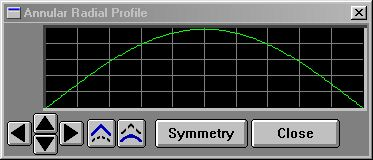10 p\$ = "annular" 20 pi = 3.141592653979382 30 for i=0 to 200 40 j = i/200 50 v = sin(j*pi)*255 60 a = SETPROF(p\$,i,v) 70 next i You'll find the names for all the system profiles located here.

 Full sinewave10 p\$ = "annular" 20 pi = 3.141592653979382 30 for i=0 to 200 40 j = (i/200)*2 50 v = ((1+sin(j*pi))/2)*255 60 a = SETPROF(p\$,i,v) 70 next i You'll find the names for all the system profiles located here.

 Upwards Ramp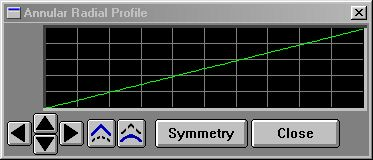10 p\$ = "annular" 20 for i=0 to 200 30 j = (i/200) 40 v = j*255 50 a = SETPROF(p\$,i,v) 60 next i You'll find the names for all the system profiles located here.

 Downwards Ramp10 p\$ = "annular" 20 for i=0 to 200 30 j = (i/200) 40 v = (1-j)*255 50 a = SETPROF(p\$,i,v) 60 next i You'll find the names for all the system profiles located here.

 Multiple Cycles of Sinewave (5)10 p\$ = "annular" 20 pi = 3.141592653979382 30 for i=0 to 200 40 j = (i/200)*2 50 v = ((1+sin(j*pi*5))/2)*255 60 a = SETPROF(p\$,i,v) 70 next i You'll find the names for all the system profiles located here.

 Using STEP() to obtain square waves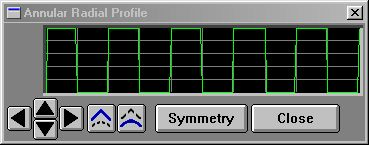10 p\$ = "annular" 20 pi = 3.141592653979382 30 for i=0 to 200 40 j = (i/200)*2 50 v = STEP((1+sin(j*pi*5))/2,.5)*255 60 a = SETPROF(p\$,i,v) 70 next i This example feeds the portion of the sinewave formula that results in a sine wave of 0 to 1 to the STEP() function, with crossing parameter of .5. That produces a result of 0 below .5, 1 above .5. You'll find the names for all the system profiles located here.

 Using SMOOTHSTEP() to obtain squarish waves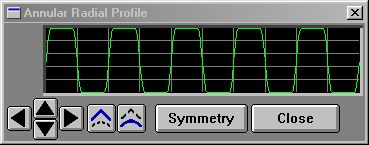10 p\$ = "annular" 20 pi = 3.141592653979382 30 for i=0 to 200 40 j = (i/200)*2 50 v = SMOOTHSTEP((1+sin(j*pi*5))/2,.3,.7)*255 60 a = SETPROF(p\$,i,v) 70 next i This example feeds the portion of the sinewave formula that results in a sine wave of 0 to 1 to the SMOOTHSTEP() function, with edge parameters of .3 and .7. That produces a result of 0 below .3, 1 above .7, and a smoothly changing value between the two. This can help you avoid the harsh edges produced by the STEP() function if it is too fierce for you. You'll find the names for all the system profiles located here.

 Using CLAMP() to limit excursions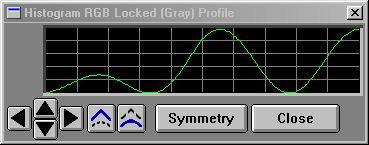200 REM makes sine wave cycles 210 c = 2.25 220 p\$ = "histo" 230 pi = 3.141592653979382 240 for i=0 to 200 250 j = (i/200)*2 260 v = ((1+sin(j*pi*c))/2)*255 270 a = SETPROF(p\$,i,v) 280 next i 290 return 300 REM rampulates from 0-1 by 1/2 profile distance 310 p\$="histo" 320 for i=0 to 200 340 a=SETPROF(p\$,i,GETPROF(p\$,i)*CLAMP((i/200)*2,0,1)) 350 next i 360 return The example image above shows what happens if you call the subroutines at 200, then at 300. The first subroutine produces a 2.25 cycle sine wave of full amplitude. The second reads that back from the profile, then multiplies each profile value by a ramp that would go linearly from 0-2 over the length of the profile ((i/200)*2) except it was fed to CLAMP() with limits of 0 and 1. So what happens is that when the ramp hits 1, it stays there, period. So the sine wave goes from 0 to full amplitude from the begining to 1/2 the profile, and after that it just stays at full amplitude. This example was taken from a metallic texture generating script that remaps linear brightness into a semblance of metallic reflections. You'll find the names for all the system profiles located here.

F/x has a series of useful scripting functions you can use to control waveforms. They are:

• CEIL()
• FLOOR()
• MIN()
• MAX()
• HILIM()
• LOWLIM()
• CLAMP()
• STEP()
• SMOOTHSTEP()
• ABS()

If you want to be a profile wizard, you'll want to look into all of these!